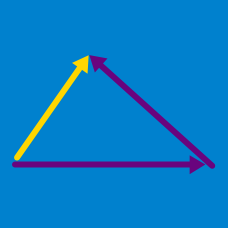Geometry

# Properties of a Vector

#### Challenge QuizzesTwo vectors $\vec{a}$, $\vec{b}$ are shown in the diagram above. Which of the following is the same vector as $\vec{a}+ \vec{b}$?Given the vectors

\begin{aligned} \vec{a} &=(1, -4),\\ \vec{b} &=(2, 3),\\ \vec{p} &=-\vec{a}+3\vec{b},\\ \vec{q} &=2\vec{a}-5\vec{b}, \end{aligned}

what is $2\vec{p}-4\vec{q}?$

What real values of $x$ and $y$ satisfy the equation $x(1,2)-y(2,3)=(5, 3)?$

Which of the following is the same vector as $\vec{c}$ in the regular hexagon below?Details and assumptions

• Let $\vec{AB}=\vec{a},$ $\vec{AC}=\vec{b},$ $\vec{AF}=\vec{c}.$Two vectors $\vec{a}$, $\vec{b}$ are shown in the diagram above. Which of the following is the same vector as $\vec{a}- \vec{b}$ ?×